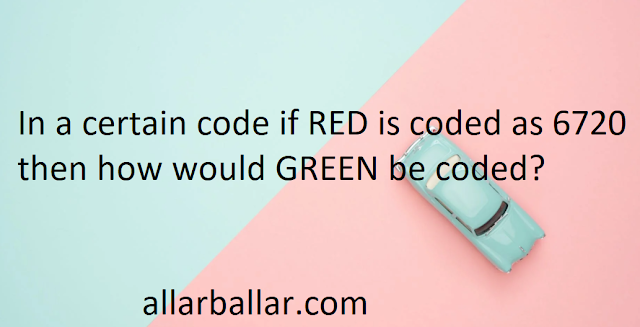# In a certain code if RED is coded as 6720 then how would GREEN be coded?Question:

In a certain code if RED is coded as 6720 then how would GREEN be coded?

Detailed Solution:

Let A=1, B=2, C=3, …………………

So, R=18, E=5, D=4, G=7, N=14

As,

RED=DER=D(4)E(5)R(18)

=D(4+2)E(5+2)R(18+2)

= D(6)E(7)R(20)

=6720

Similarly,

GREEN=NEERG

=N(14)E(5)E(5)R(18)G(7)

=N(14+2)E(5+2)E(5+2)R(18+2)G(7+2)

=N(16)E(7)E(7)R(20)G(9)

=1677209

Therefore, In a certain code if RED is coded as 6720 then how would GREEN be coded as 1677209.